## Visualization of Cytometry data using the new `ggCyto` package.

The `ggCyto` package was developed at the RGLab by Mike Jiang. It is new and under active development.
Here we aim to demonstrate some of its functionality.

By overloading `ggplot`’s `fortify` method, we make cytometry data fully compatible with `ggplot`.

#### Subsetting the data

It usually doesn’t make sense to visualize 100’s or FCS samples, so we’ll subset the data for visualization, restricting ourselves to the first two subjects. We can use `subset` on the GatingSet object to subset by `pData` variables. This is pretty standard `BioConductor` behavior.

``````# Subset the data for a demo of visualization.
ptids <- unique(pData(tbdata)[["PID"]])[1:2]
tbdata <- subset(tbdata, `PID` %in% ptids)
Rm("CD4",tbdata)``````

Furthermore, we’ll focus on the CD3+ cell subsets for this demonstration. These are extracted into a `flowSet`.

``````# extract the CD3 population
fs <- getData(tbdata, "CD3")``````

### ggcyto + flowSet

#### 1-dimensional plots - histograms.

The simplest way to visualize FCM data is via one-dimensional histograms or density plots. This is supported using the standard `ggplot2` `geom_xxxx` interface.

Here we specify that we want a histogram, and we map the aesthetic `x` to the variable `CD4`, which corresponds to the dimension/marker we want to plot.

`ggCyto` automatically facets by the `name` variable, which usually represents individual FCS files.

``````p <- ggcyto(fs, aes(x = CD4))
p1 <- p + geom_histogram(bins = 60)
p1``````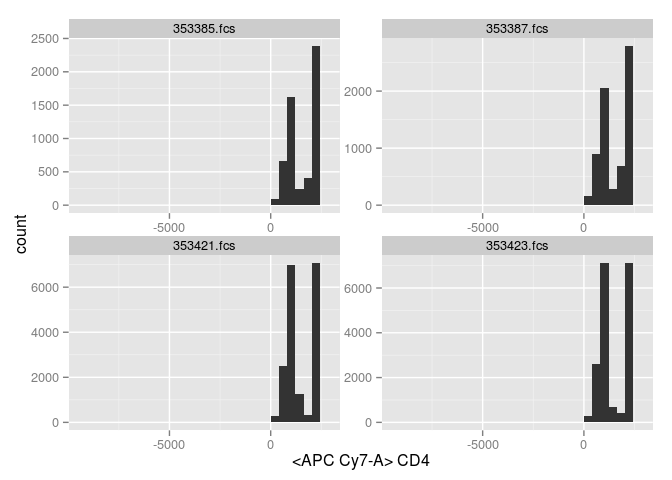#### Change the limits to reflect the instrument range.

`ggCyto` will show you the full range of the data, which is often more than the instrument range. We can restrict the range to the instrument range, using `ggcyto_par_set`.

Valid values are `data` and `instrument`.

``````myPars <- ggcyto_par_set(limits = "instrument")
p1 + myPars``````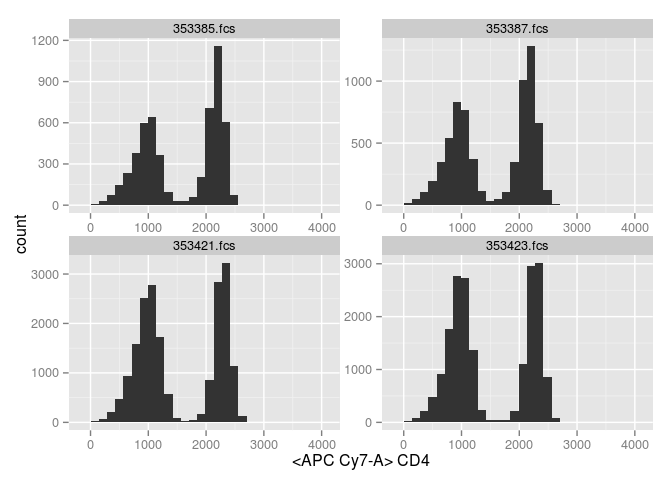#### View the default parameter settings

We can print the default parameter settings using `ggcyto_par_default`.

``````# print the default settings
ggcyto_par_default()``````
``````## \$limits
##  "data"
##
## \$facet
## facet_wrap(name)
##
## \$hex_fill
## continuous_scale(aesthetics = "fill", scale_name = "gradientn",
##     palette = gradient_n_pal(colours, values, space), na.value = na.value,
##     trans = "sqrt", guide = guide)
##
## \$lab
## \$labels
##  "both"
##
## attr(,"class")
##  "labs_cyto"
##
## attr(,"class")
##  "ggcyto_par"``````

#### Density plot - 1D

Of course, other geometries are supported. `geom_density` will generate a denstiy plot rather than a histogram.

``````p = p + geom_density() +  geom_density(fill = "black") + myPars
p``````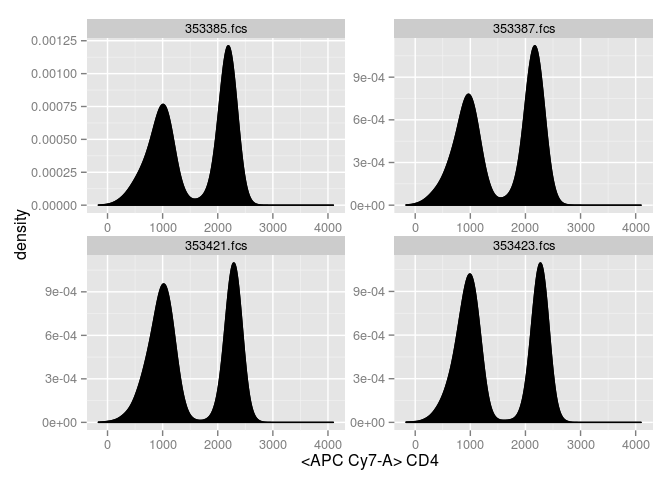### Facetting is also supported.

As you saw, the default faceting is using the `name` variable. But, any variable defined in the `pData` slot of the `flowSet` is valid.

``kable(pData(fs))``
Peptide Stim PID EXPERIMENT NAME name known_response
353385.fcs DMSO General 01-0917 130517_TB-ICS_ACS_GP 353385.fcs non-responder
353387.fcs ESAT-6 General 01-0917 130517_TB-ICS_ACS_GP 353387.fcs non-responder
353421.fcs DMSO General 01-0996 130517_TB-ICS_ACS_GP 353421.fcs responder
353423.fcs ESAT-6 General 01-0996 130517_TB-ICS_ACS_GP 353423.fcs responder

Here we facet by Peptide stimulation and known_response (which comes from previous analysis).

``````#change facetting (default is facet_wrap(~name))
p + facet_grid(known_response ~ Peptide)``````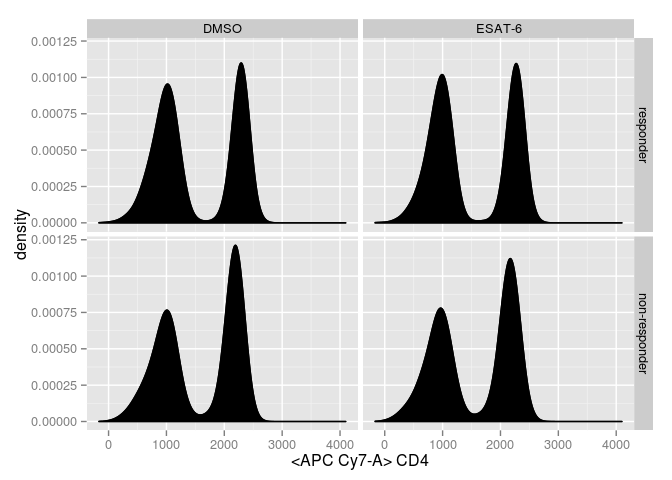#### 2-dimensional dot plots

The typical view of FCM data is using two-dimensional dot plots. Hexagonal binning is a popular and rapid was to view the data.

Again, axis limits need to be specified since by default `ggcyto` will present all the data, includig outliers that have unusually large positive or negative values.

``````# 2d hexbin
p <- ggcyto(fs, aes(x = CD4, y = CD8)) + geom_hex(bins = 60) + ylim(c(-100,4e3)) + xlim(c(-100,3e3))
p``````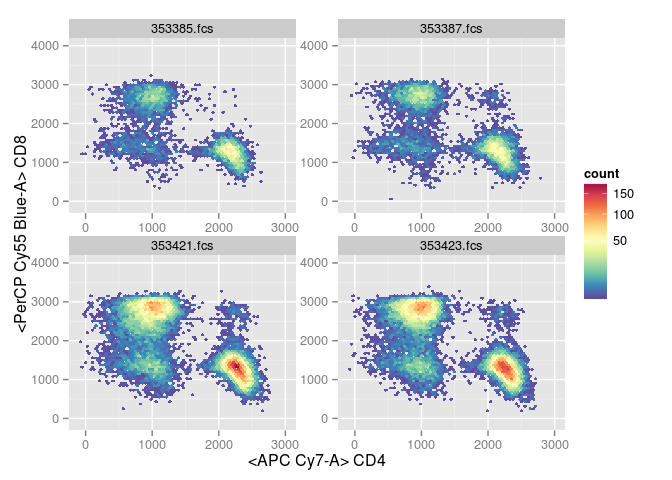#### Changing the color scale

The default colour scale can be changed using `scale_fill_gradient`.

For example, a color brewer scale using the `PiYG` scale, with a square root transform of the counts.

``p + scale_fill_gradientn(colours = brewer.pal(n=8,name="PiYG"),trans="sqrt")``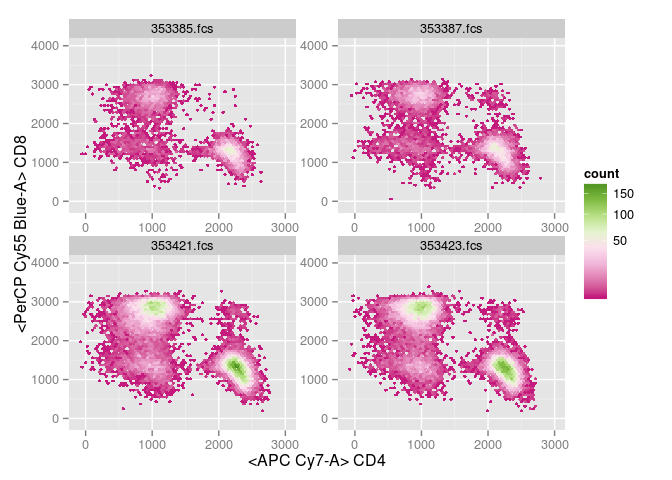Or grayscale.

``p + scale_fill_gradient(trans = "sqrt", low = "gray", high = "black")``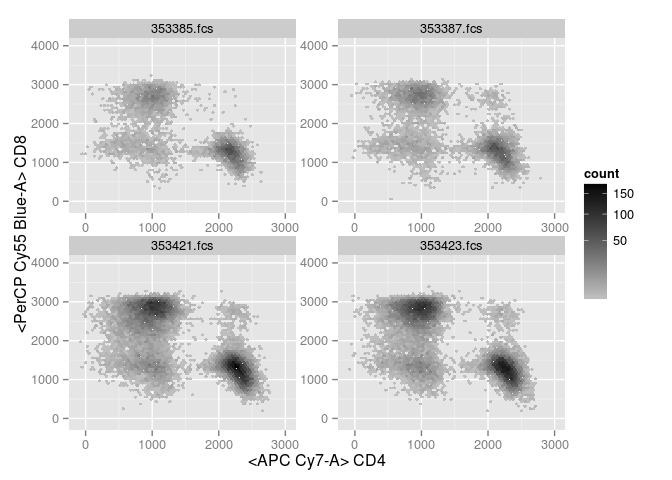### Contours

`geom_density2d` behaves as expected.

``ggcyto(fs, aes(x = CD4, y = CD8))+ geom_hex(bins = 60)+geom_density2d(colour = "black")+ylim(c(-100,4e3)) + xlim(c(-100,3e3))  ``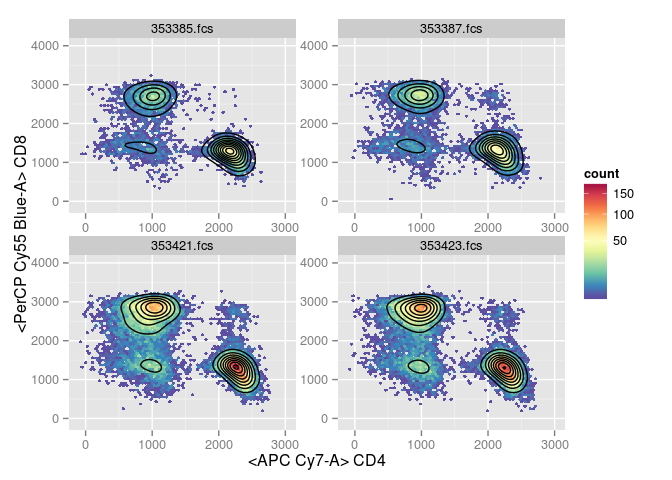### Plotting gates for flowSet objects

It’s possible to plot gates on top of the data.

One way to do so is to extract the gate from the `GatingSet` and add it explicitly.

``````# add geom_gate layer
p <- ggcyto(fs, aes(x = CD4, y = CD8)) + geom_hex(bins = 60) + ylim(c(-100,4e3)) + xlim(c(-100,3e3))
g <- getGate(tbdata, "CD4+")
p <- p + geom_gate(g)
p``````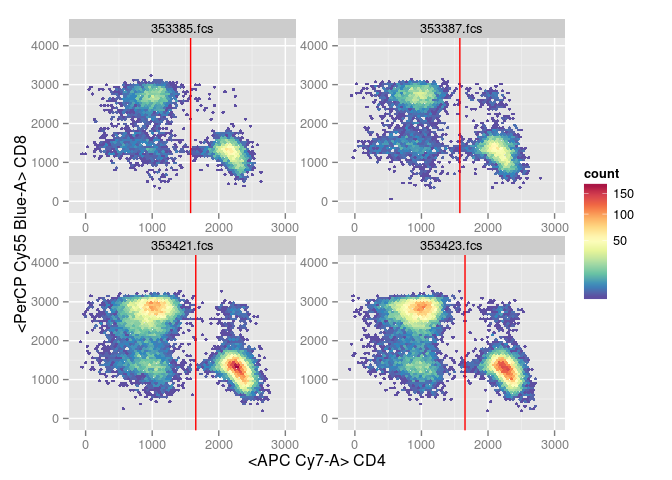Overlay statistics for cell populations.

``````# add geom_stats
p + geom_stats()``````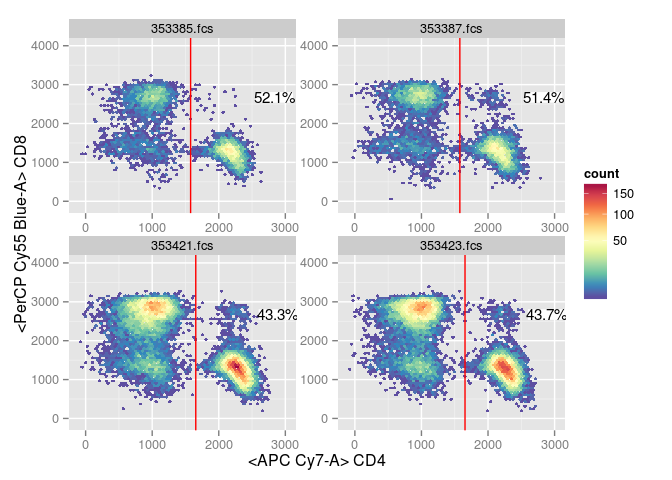### Use a GatingSet rather than flowSet

#### ggcyto + GatingSet

As before, but we can use a GatingSet object rather than a flowSet. Again, dimensions are mapped to aesthetics using marker names.

``````#use customized range to overwrite the default data limits
myPars <- ggcyto_par_set(limits = list(y = c(-100,4e3), x = c(-100,3e3)))
p <- ggcyto(tbdata, aes(x = CD4, y = CD8), subset = "CD3")
p <- p + geom_hex(bins = 64) + myPars
p``````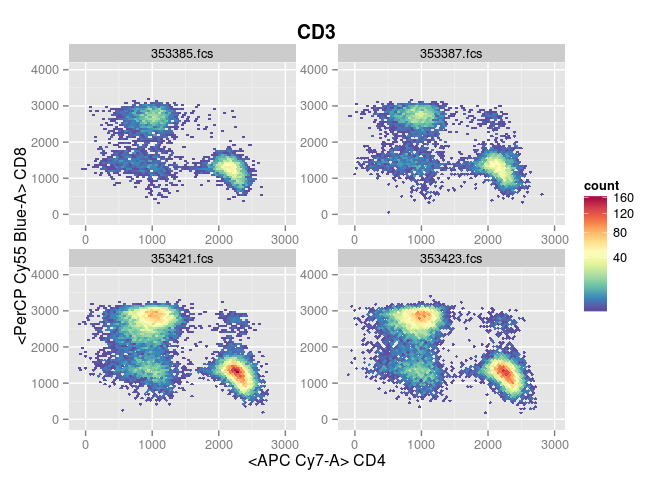If we want to use marker names on the axes rather than channel and marker names, that is possible.

``````#only display marker on axis
p <- p + labs_cyto("marker")
p``````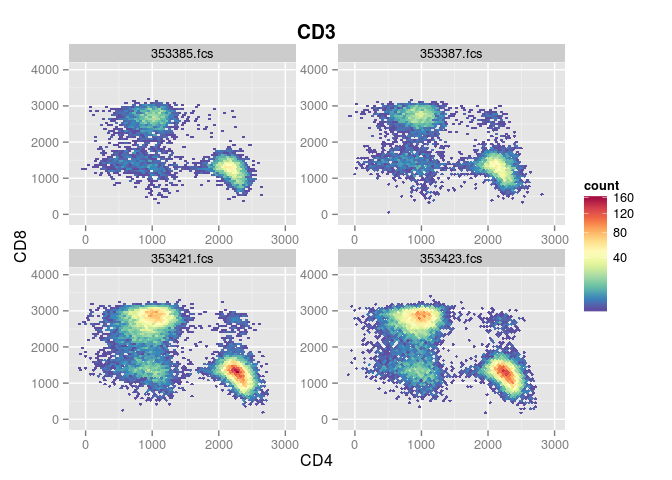#### Plotting gates for GatingSet objects

When plotting gates, we don’t need to extract them explicitly as they’re part of the object.

One gate.

``````# add gate
p + geom_gate("CD4+CD8-")``````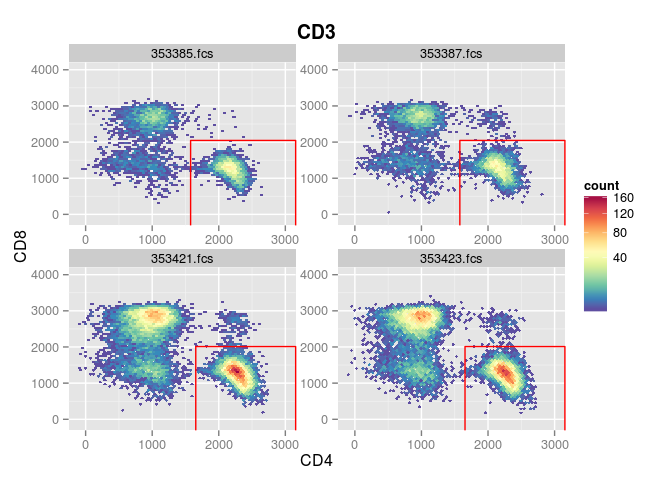Two gates.

``````# add two gates
p <- p + geom_gate(c("CD4+CD8-","CD4-CD8-"))
p``````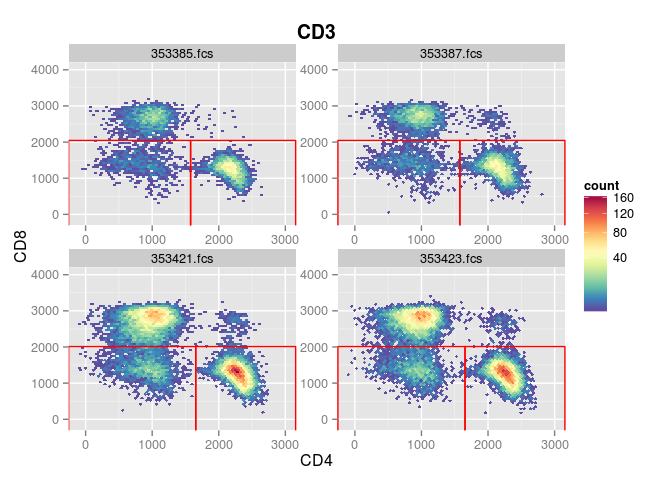Overlay population statistics.

``p + geom_stats() ``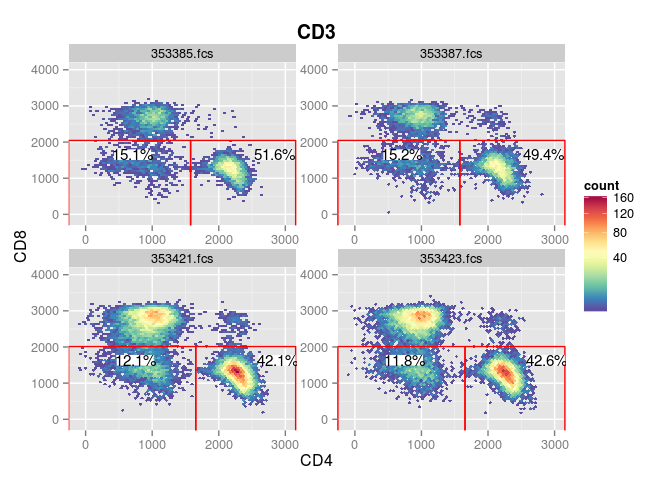Overlay population statistics for just one population.

``````# add stats just for one specific gate
p + geom_stats("CD4+CD8-")``````